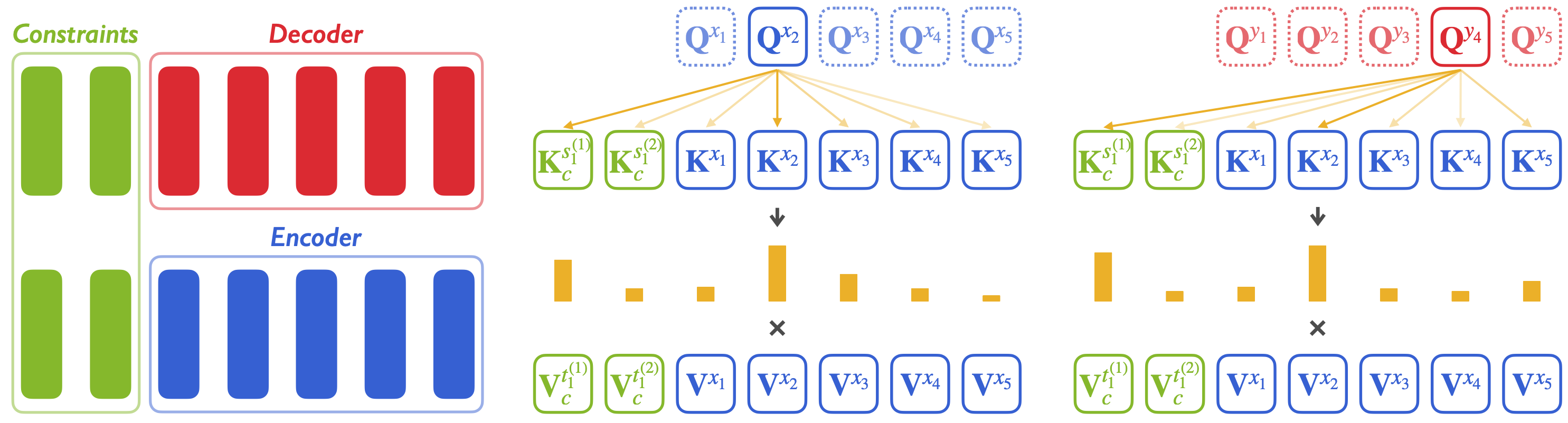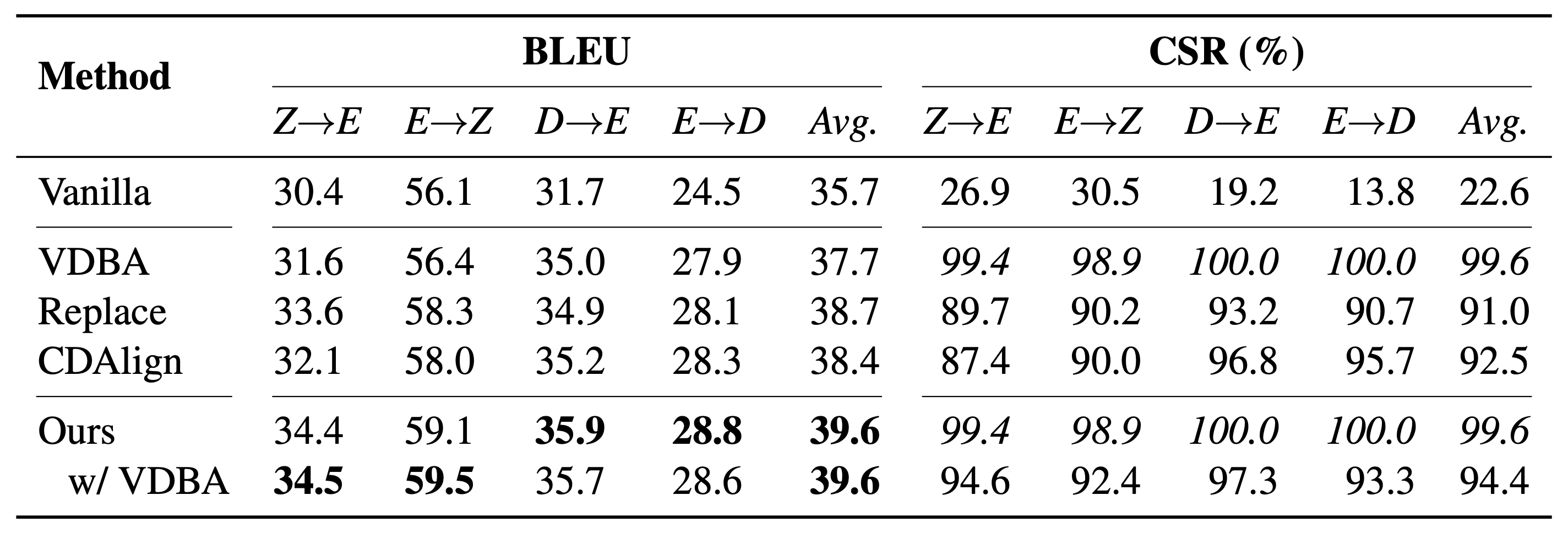Lexical translation error is an important kind of error that current neural machine translation (NMT) models suffer from. To this end, several researchers direct their attention to lexically constrained NMT, which aims to control the translation of some specific source-side terms. Lexically constrained NMT can be applied to many scenarios, including entity translation, interactive machine translation, and so on. However, due to the end-to-end nature of modern NMT models (Post et al., 2018), it is not such straightforward to impose the pre-specified lexical choice into the translation process of NMT models. Previously, some studies propose to modify the beam search algorithm to explicitly introduce the lexical constraints during inference (Hokamp et al., 2017; Post et al., 2018; Hu et al., 2019). Some other works instead leave the model and the algorithm mostly unchanged and directly insert the target-language constraints to the input sentence (Song et al., 2019; Chen et al., 2020). The recent progress triggers us to raise a research question: can we integrate the lexical constraints through architecture design?

We revisited each component of the Transformer model and found that the attention mechanism is like the model looking up a “dictionary”, whose keys and values are both continuous vectors, whereas the human dictionaries consist of discrete keys and values. Therefore, we propose to add lexical constraints into the attention modules to guide the Transformer model in our ACL 2022 paper.

## Problem Definition

For a source-language sentence $$\mathbf{x}$$ and a set of constraints $$\{\langle \mathbf{u}^{(n)}, \mathbf{v}^{(n)}\rangle\}_{n=1}^{N}$$, we expect the lexically constrained translation system to translate each $$\mathbf{u}^{(n)}$$ into $$\mathbf{v}^{(n)}$$. In this work, we propose to vectorize $$\{\langle \mathbf{u}^{(n)}, \mathbf{v}^{(n)}\rangle\}_{n=1}^{N}$$ into continuous vectors and then integrate them into the Transformer model.

## ApproachAs illustrated in the above figure, we map lexical constraints into a series of continuous vectors, which are then prepended before the original keys and values of the attention module. Specifically, the source-language constraints are prepended before the keys while the target-language constraints are prepended before the values. For instance, if we specify a constraint “Beatles -> 甲壳虫”, then “Beatles” are firstly mapped into continuous vectors and then prepended to the attention keys, and similarly “甲壳虫” are vectorized and prepended to the attention values. We integrate the constraints to the self-attention modules in the encoder and the cross-attention modules in the decoder.

Since some constraints contains more than more tokens at each side and the individual tokens are not always monotonically aligned, we need to preprocess the constraints before provide them to the attention modules. Specifically, we use a multi-head attention layer to align the bilingual constraints. Moreover, some recent studies find that the representation evolves from the first layer to the last layer inside the Transformer model. To this end, we use an independent projection module to adapt the constraints into the corresponding manifolds for each specific layer. We also use a pointer network to further encourage the appearance of the pre-specified constraints. Please refer to our paper for more details.

## ResultsThe above table shows the experimental results of our method. We compare our approach with three representative baselines:

• VDBA (Hu et al., 2019), which is an improved constrained decoding algorithm;
• Replace (Song et al., 2019), which is an effective data augmentation method;
• CDAlign (Chen et al., 2021), which is the most recent lexically constrained method when we conduct our experiments.

Since our approach only modifies the model architecture, our model can also employ VDBA as our decoding algorithm. The results demonstrate the effectiveness of our model. Surprisingly, even without VDBA, our method can also achieve 94.4% CSR (copying success rate), which means that 94.4% of the pre-specified constraints are successfully generated by our model. Please refer to the paper for more results.

## What’s Next ?

Existing lexically constrained approaches can be roughly divided into three categories: constrained decoding, data augmentation, and architecture design. Although constrained decoding can guarantee the appearance of the constraints, its speed is significantly slower than vanilla beam search. Data augmentation and architecture design both do not significantly slow down the inference procedure, but these methods can not achieve 100% CSR. In our recent work, we propose a template-based method that can achieve 100% CSR with a speed that is comparable with the vanilla beam search. We suggest to read this template-based approach for researchers who are interested in lexically constrained translation :)